• ## LaTex中文模板

万次阅读 2016-03-06 15:25:31
LaTex中文模板一份之前想在网上找一份通用的latex模板来写文档，省去写导言等的时间。后来发现，网上的模板要么太复杂，要么一运行就出错，这里我提供一份自己常用的中文模板。一些宏包我以注释的形式给出，当需要的...
LaTex中文模板一份

之前想在网上找一份通用的latex模板来写文档，省去写导言等的时间。后来发现，网上的模板要么太复杂，要么一运行就出错，这里我提供一份自己常用的中文模板。一些宏包我以注释的形式给出，当需要的时候，将其释放即可。

\documentclass[a4paper]{ctexart} %CTEX报告文章格式

\usepackage[top=3cm,bottom=2cm,left=2cm,right=2cm]{geometry} % 页边距
%\usepackage{amsmath} %数学公式
\usepackage{amsthm}
%\usepackage{longtable} %长表格
\usepackage{graphicx} %图片
%\usepackage{tikz} %画图
%\usepackage{cite}
%\usepackage{listings}
%\usepackage{amsfonts}
%\usepackage{subfigure}
%\usepackage{float}
%\usepackage[colorlinks,linkcolor=black,hyperindex,CJKbookmarks,dvipdfm]{hyperref}
%\lstset{language=Mathematica}%这条命令可以让LaTeX排版时将Mathematica键字突出显示
%\lstset{extendedchars=false}%这一条命令可以解决代码跨页时，章节标题，页眉等汉字不显示的问题
%\usetikzlibrary{shapes,arrows} %tikz图形库
%\usepackage{overpic} %图上标记
%\usepackage{ccaption} %中英文题注
%\usepackage[numbers,sort&compress]{natbib} %参数代表：数字和排序与压缩
%\bibliographystyle{GBT7714-2005NLang} %参考文献格式设为GBT7714-2005N.bst
%\usepackage[draft=false,colorlinks=true,CJKbookmarks=true,linkcolor=black,citecolor=black,urlcolor=blue]{hyperref} %参考文献跳转，此宏包会自动载入graphicx
%\usepackage{textcomp} %摄氏度符号
%\usepackage{ccmap} %pdf中文复制
%\usepackage{myfont} %字体
%\usepackage{color} %gnuplot彩色文字
%\usepackage{texshade} %texshade，此宏包与graphicx冲突，故放最后
% \usepackage{indentfirst}
% \setlength{\parindent}{2em}
%\makeatletter
%%\renewcommand{\chapter} {\endgraf
%%   \thispagestyle{empty}           % Page style of chapter page is 'plain'
%%   \global\@topnum\z@              % Prevents figures from going at top of page.
%%   \@afterindenttrue               % Inserts indent in first paragraph.  Change
%%   \secdef\@chapter\@schapter}     % to \@afterindentfalse to remove indent.
%\makeatother

%\renewcommand{\textfraction}{0.15}
%\renewcommand{\topfraction}{0.85}
%\renewcommand{\bottomfraction}{0.65}
%\renewcommand{\floatpagefraction}{0.60}
%\author{陆嵩}

\begin{document}
%\CTEXoptions[contentsname={\bfseries\zihao{4} 目\quad 录}]
%%\CTEXsetup[nameformat+={\zihao{3}}]{chapter}
%%\CTEXsetup[titleformat+={\zihao{3}}]{chapter}
%\CTEXsetup[number={\arabic{chapter}}]{chapter}
%\CTEXsetup[name={,}]{chapter}
%\CTEXsetup[format={\zihao{4}}]{section}
%\CTEXsetup[format={\bfseries\zihao{4}}]{subsection}
%\CTEXsetup[format={\bfseries\zihao{-4}}]{paragraph}
%%\CTEXsetup[beforeskip={0em}]{paragraph}
%\CTEXsetup[beforeskip={0pt}]{chapter}
%\CTEXsetup[afterskip={2em}]{chapter}
%%\CTEXsetup[afterskip={0pt}]{subsection}
%%\captionwidth{0.8\textwidth}
%%\changecaptionwidth
%\thispagestyle{empty}
%%%\pagestyle{plain}
%%\newpage
%\setcounter{page}{1}
%\pagenumbering{Roman}
%\noindent\addcontentsline{toc}{section}{摘要}
\begin{center}\zihao{3}\textbf{牛顿方法在多项式求根方面的应用}\end{center}
\begin{center}\zihao{5}\textbf{郑州大学\ 数学与统计学院 \ 信息与计算科学 \ 陆嵩}\end{center}
%\begin{center}\zihao{4}\textbf{摘要}\quad\zihao{-4}\end{center}
%\vspace{1em}
%%\noindent\zihao{4}\textbf{关键词}\quad\zihao{-4} 最小旋转曲面；Mathematica；样条插值；变分法
%%\tableofcontents
%\setcounter{page}{1}
%\pagenumbering{arabic}
%\pagestyle{headings}

%5.5到5.8节主要介绍了了多项式求根和一些典型的求根方法。其中有很多我们想不到的方法。比如说，正如我们看到的，Bauer（1956）、Jenkins和Traub（1970）、Nickel（1966）以及Henrici（1974），这些人提到了一些。
%
%我们确定一般多项式的根的一般方法的重要性有时会被评价过高。在实际应用中的多项式大部分会以一种特定的形式给定，比如说，特征多项式就是这样。之后，你将学习到，多项式的根就是矩阵的特征值，进一步，我们将在第六章详细描述这个方法。
%
%我们将详细讲述牛顿方法在寻找给定多项式$p(x)$的根方面的应用。为了评价牛顿迭代方程，
%${x_{k{\rm{ + }}1}}: = {x_k} - \frac{{p({x_k})}}{{p'({x_k})}}$
%我们不得不计算多项式以及多项式一阶导在点$x=x_k$ 处的值。假定多项式以下列的形式给出
%$p(x) = {a_0}{x^n} + {a_1}{x^{n - 1}} + \cdots + {a_n}$
%那么$p(x_k)$和$p'(x_k)$可用如下方法计算：对于$x = \xi$,
%$p(\xi ) = ( \cdots (({a_0}\xi + {a_1})\xi + {a_2})\xi + \cdots )\xi + {a_n}$.
%对于在这个表达式中乘数$\xi$，可用如下的递归格式
%\begin{equation}
%\label{5.5.1}
%\begin{array}{l}
%{b_0}: = {a_0}\\
%{b_i}: = {b_{i - 1}}\xi  + {a_i},i = 1,2,...,n.
%\end{array}
%\end{equation}
%多项式$p$在$\xi$出的值可以这样给定：
%$p(\xi ) = {b_n}$
%
%通过递归式子(\ref{5.5.1})评估多项式的算法被称作 Horner方法。我们所能得到的$b_i$的量，也正是以下多项式的的系数
%${p_1}(x): = {b_0}{x^{n - 1}} + {b_1}{x^{n - 2}} + ... + {b_{n - 1}}$
%用$x-\xi$去除$p(x)$，我们能得到：
%\begin{equation}\label{5.5.2}
%p(x) = (x - \xi )p{}_1(x) + {b_n}
%\end{equation}
%这通过比较式(ref{5.5.2})两边的系数，很容易被证实。进一步，对式(ref{5.5.2})关于$x$两边求导，并让$x=\xi$,我们得到
%$p'(\xi ) = {p_1}(\xi )$
%因此，一阶导数$p'(\xi)$能通过重复使用Horner方法来确定，用前一个的结果来做后一个式子的系数。
%$p'(\xi ) = ( \cdots ({b_0}\xi + {b_1})\xi {\rm{ + }} \cdots )\xi + {b_{n - 1}}$
%
%通常地，多项式$p(x)$一般是以除此之外的其他一些格式给出的，
%$p(x) = {a_0}{x^n} + {a_1}{x^{n - 1}} + \cdots + {a_n}$
%特别重要一种情况是，$p(x)$正好是三对角矩阵的特征多项式
%$J = \left[ {\begin{array}{*{20}{c}} %{{\alpha _1}}&{{\beta _2}}&{}&0\\ %{{\beta _2}}& \ddots & \ddots &{}\\ %{}& \ddots & \ddots &{{\beta _n}}\\ %0&{}&{{\beta _n}}&{{a_n}} %\end{array}} \right]$
%其中，${\alpha _i},{\beta _i}$是实数。
%也就是说，多项式是特征矩阵
%${p_i}(x) = \det \left( {\left[ {\begin{array}{*{20}{c}} %{{\alpha _1} - x}&{{\beta _2}}&{}&0\\ %{{\beta _2}}& \ddots & \ddots &{}\\ %{}& \ddots & \ddots &{{\beta _i}}\\ %0&{}&{{\beta _i}}&{{a_i} - x} %\end{array}} \right]} \right)$
%原则上的顺序主子式。我们有递推序列:
%\begin{equation}
%\begin{array}{l}\label{5.5.3}
%{p_0}(x): = 1\\
%{p_1}(x): = ({\alpha _1} - x) \cdot 1\\
%{p_i}(x): = ({\alpha _i} - x){p_{i - 1}}(x) - {\beta _i}^2{p_{i - 2}}(x)\;\;\;\;\;\;\;\;\;\;i = 2,3, \cdots n.\\
%p(x): = det(J - xI): = {p_n}(x)
%\end{array}
%\end{equation}
% 这些公式能够被用来计算对于任意$x=\xi$的$p(\xi)$，和任意给定的矩阵元素$\alpha_i,\beta_i$。类似地，我们通过对式(\ref{5.5.3}) 求导，可以推得$p'(x)$的递推方程如下：
%\begin{equation}\label{5.5.4}
%\begin{array}{l}
%{p_0}'(x): = 0\\
%{p_1}'(x): =  - 1\\
%{p_i}'(x): =  - {p_{i - 1}}(x) + ({\alpha _i} - x)p{'_{i - 1}}(x) - {\beta _i}^2p{'_{i - 2}}(x)\;\;\;\;\;\;\;\;\;\;i = 2,3, \cdots n.\\
%p'(x): = p{'_n}(x)
%\end{array}
%\end{equation}
%两个递推公式(\ref{5.5.3})和(\ref{5.5.4})能够同时被计算。
%
%通过5.3节对牛顿方法一般性的讨论，我们清楚地知道，当且仅当初始点$x_0$足够接近零点$\xi$的时候，由牛顿方法确定的$x_k$是收敛的。一个糟糕的初始值可能会导致序列$x_k$发散，即使是对多项式也不例外。如果实多项式$p(x)$没有实根（例如：$p(x)=x_2+1$），那么牛顿方法对于任意的实数域内的初始值是不可能收敛的。对于任意的多项式个例，我们没有通用而保险的选取有效初值的方法。但是呢，在一种重要而特别的情况下，我们确实存在一种通用的方法。也就是这种情况，如果所有的根$\xi$是实的($i=1,2,...,n.)$)，并且满足：
%${\xi _1} \ge {\xi _2} \ge \cdots \ge {\xi _n}.$
%在5.6部分，定理（5.6.5）将向我们证明，那么由式(\ref{5.5.3})定义的多项式在矩阵元素$\alpha_i,\beta_i$是实数的前提下将具备这种属性。
%
%\newtheorem{theorem}{Theorem}
%\begin{theorem}\label{5.5.5}
%$p(x)$是维度$n \ge 2$的实系数多项式，如果对于所有的根$\xi_i$都是实数，其中，
%${\xi _1} \ge {\xi _2} \ge \cdots \ge {\xi _n}.$
%那么，牛顿方法对于任意的初值$x_0 \ge \xi_1$,都能产生一个严格递减的序列$x_k$.
%\end{theorem}
%\begin{proof}[证明]
%不失一般性，我们可以假设$p(x_0) > 0$
%既然$p(x_0)$对于$x>\xi_1$不换号，那么对于任意的$x>\xi_1$我们有，
%$p(x) = {a_0}{x^n} + \cdots + {a_n} > 0$
%因此，我们可以知道$a_0>0$.并且通过罗尔定理，我们知道，导数$p'(x)$有$n-1$个实根，并且
%${\xi _1} \ge {\alpha _1} \ge {\xi _2} \ge {\alpha _2} \ge \cdots \ge {\alpha _{n - 1}} \ge {\xi _n}$
%因为$p'$的维度是$n-1$且大等于1，上面表示的$\alpha_i$恰好是它全部的根。因$a_0>0$容易推知，对于任意的$x>\alpha_1$，我们有$p'(x)>0$。再次运用罗尔定理，和重新使用条件$n \ge 2$,我们可以知道
%\begin{equation}\label{5.5.6}
%\begin{array}{l}
%p''(x) > 0\;\;\;x > \alpha {}_1\\
%p'''(x) \ge 0\;\;\;x \ge \alpha {}_1
%\end{array}
%\end{equation}
%因此，对于任意的$x \ge \alpha_1$,$p,p'$都是下凸函数。
%
%
%现在，由于$p'(x_k)>0 ,p(x_k)>0$$x_k>\xi_i$暗示着
%${x_{k + 1}} = {x_k} - \frac{{p({x_k})}}{{p'({x_k})}} < {x_k}$
%现在还有待证明的是，我们用牛顿方法不能“过调”。从式（\ref{5.5.6}）,我们知道$x_k>\xi_1 \ge \alpha_1$，进一步，由泰勒定理，我们可以推导得到
%$0 = p({\xi _1}) = p({x_k}) + ({\xi _1} - {x_k})p'({x_k}) + \frac{1}{2}{({\xi _1} - {x_k})^2}p''(\delta ) > p({x_k}) + ({\xi _1} - {x_k})p'({x_k})\;\;\;{\xi _1} < \delta < {x_k}$
%由$x_{k+1}$的定义式，移向我们可以得到$p(x_k)=p'(x_k)(x_k-x_k+1)$，因此，
%$0 > p'({x_k})({x_k} - {x_{k + 1}} + {\xi _1} - {x_k}) = p'({x_k})({\xi _1} - {x_{k + 1}})$
%再由$p'(x_k)>0$，立得$x_k+1>\xi_1$
%\end{proof}
%
%为了之后的使用，我们注意定理\ref{5.5.6}接下来的结果:
%\newtheorem{lemma}{Lemma}
%\begin{lemma}\label{5.5.7}
%设$p(x) = {a_0}{x^n} + {a_1}{x^{n - 1}} + \cdots + {a_n}>0,$ 是一个维度$n \ge 2$且所有根都是实数的实系数多项式。假如$\alpha_1$是$p'$ 最大的根，那么对于任意的$x \ge \alpha_1$，有$p'''(x) \ge 0$,换言之，对于任意的$x \ge \alpha_1$，$p'$是一个下凸函数。
%\end{lemma}
%
%
%我们仍然面临一个问题。那就是在事前不知道$\xi_1$ 的前提下，如何寻找一个比$\xi_1$大的初值$x_0$呢？以下的定理可以解决这个问题：
%\begin{theorem}\label{5.5.8}
%对任意的一个多项式$p(x) = {a_0}{x^n} + {a_1}{x^{n - 1}} + \cdots + {a_n}$，它的所有根$\xi$都满足：
%$\left| {{\xi _i}} \right| \le \max \left\{ {\left| {\frac{{{a_n}}}{{{a_0}}}} \right|,1 + \left| {\frac{{{a_{n - 1}}}}{{{a_0}}}} \right|, \cdots ,1 + \left| {\frac{{{a_1}}}{{{a_0}}}} \right|} \right\}$
%$\left| {{\xi _i}} \right| \le \max \left\{ {1,\sum\limits_{j = 1}^n {\left| {\frac{{{a_j}}}{{{a_0}}}} \right|} } \right\}$
%$\left| {{\xi _i}} \right| \le \max \left\{ {\left| {\frac{{{a_n}}}{{{a_{n - 1}}}}} \right|,2\left| {\frac{{{a_{n - 1}}}}{{{a_{n - 2}}}}} \right|, \cdots ,2\left| {\frac{{{a_1}}}{{{a_0}}}} \right|} \right\}$
%$\left| {{\xi _i}} \right| \le \sum\limits_{j = 0}^{n - 1} {\left| {\frac{{{a_{j + 1}}}}{{{a_j}}}} \right|}$
%$\left| {{\xi _i}} \right| \le 2\max \left\{ {\left| {\frac{{{a_1}}}{{{a_0}}}} \right|,\sqrt {\left| {\frac{{{a_2}}}{{{a_0}}}} \right|} ,\sqrt{{\left| {\frac{{{a_3}}}{{{a_0}}}} \right|}} \cdots ,\sqrt[n]{{\left| {\frac{{{a_n}}}{{{a_0}}}} \right|}}} \right\}$
%\end{theorem}
%
%这些差异中的一部分将在6.9节中证明，同时也和Househoder(1970)做一个比较。其他的不同将在Marden(1949)能找到。
%
%二次收敛并不意味着快速收敛。如果选取的初值离根非常远的话，那么牛顿方法在开始的时候可能就收敛得非常缓慢。事实上，如果$x_k$足够大，那么
%${x_{k + 1}} = {x_k} - \frac{{x_k^n + \cdots }}{{nx_k^{n - 1} + \cdots }} \approx {x_k}\left( {1 - \frac{1}{n}} \right)$
%导致在$x_k$和$x_{k+1}$之间变化非常小。这些结果导致我们去寻求更好的“双步法”来代替直接的牛顿方法，如下：
%${x_{k + 1}} = {x_k} - 2\frac{{p({x_k})}}{{p'({x_k})}}\;\;\;k = 0,1,2, \ldots$
%
%
%当然，现在我们有了“过调”的风险。特别地，在多项式只有实根，且初值$x_0 \ge \xi_1$的情况下，迭代出的某些$x_{k+1}$可能会越过最大一个根$\xi_1$,从而失去了定理\ref{5.5.5}的优势。但是不要怕，这种“过调”导致的不收敛是可以被消除的。由于多项式的一些优良属性，在上述情况发生的前提下，我们可以找到一个好的新初值$y(\xi_1 \ge > \xi_2)$，接着用牛顿方法进行迭代，最后也能收敛。之后将给出以下定理的结果：
%\begin{theorem}\label{5.5.9}
%设$p(x) = {a_0}{x^n} + {a_1}{x^{n - 1}} + \cdots + {a_n}>0,$ 是一个维度$n \ge 2$且所有根都是实数的实系数多项式。并且$p(x)$ 的根排序如下，${\xi _1} \ge {\xi _2} \ge \cdots \ge {\xi _n}.$ 另外，假如$\alpha_1$是$p'$最大的根，
%${\xi _1} \ge {\alpha _1} \ge {\xi _2}$
%对于$n=2$,我们需要特别要求$\xi_1 \ge \xi_2$，那么对于每一个$z>\xi_1$,一些符号的定义如下：
%$z': = z - \frac{{p(z)}}{{p'(z)}},y: = z - 2\frac{{p(z)}}{{p'(z)}},y': = y - \frac{{p(y)}}{{p'(y)}}$
%（图\ref{Figure 8}很好地刻画了这个问题），则有以下结论：
%$\begin{array}{l}\label{5.5.10} %{\alpha _1} < y\\ %{\xi _1} \le y' \le z' %\end{array}$
%\begin{figure}[!h]
%\small
%\centering
%\includegraphics[width=12cm]{111.eps}
%\caption{Geometric interpretation of the double-step method} \label{Figure 8}
%\end{figure}
%\end{theorem}
%容易证明当$n=2$并且$\xi_1=\xi_2$时，也就意味着对于任意的$z>\xi_1$,都有$y=\xi_1$
%\begin{proof}[证明]
%再次假设当$z>\xi_1$时，$p(z)>0$。对于这样一个$z$值，我们假设有两个两个量$\Delta_0,\Delta_1$(如图\ref{Figure 8}所示)定义如下：
%${\Delta _0}: = p(z') = p(z') - p(z) - (z' - z)p'(z) = \int_z^{z'} {\left[ {p'(t) - p'(z)} \right]} dt$
%${\Delta _1}: = p(z') - p(y) - (z' - y)p'(y) = \int_y^{z'} {\left[ {p'(t) - p'(y)} \right]} dt$
%当然，这两个量还可以分别地被解释为关于$p'(x)$的图上的两块区域，见图\ref{Figure 9}。
%\begin{figure}[!h]
%\small
%\centering
%\includegraphics[width=12cm]{222.eps}
%\caption{The quantities $\Delta_0$ and $\Delta_1$ interpreted as areas} \label{Figure 9}
%\end{figure}
%通过引理\ref{5.5.7},$p'(x)$在$x \ge \alpha_1$是个下凸函数。因此，我们知道$z'-y=z-z'>0$(由定理\ref{5.5.5}知道它是正值)，我们可以得到
%\begin{equation}\label{5.5.11}
%{\Delta _1} \le {\Delta _0}\;\;\;y \ge {\alpha _1}
%\end{equation}
%$\Delta_0=\Delta_1$当且仅当$p'$是线性函数，也就是说$p$是次数为2的多项式。现在我们分为$y>\xi_1,y=\xi_1,y<\xi_1$三种情况来讨论。对于$y>\xi_1$,由定理\ref{5.5.5},立证得。对于$y=\xi_1$,我们首先证明$\xi_2<\alpha_1<\xi_1$,也就是说$\xi_1$是$p$的单根。假如$y=\xi_1=\xi_2=\alpha_1$是复根，那么，通过$n \ge 3$的假设，由式\ref{5.5.11},我们能得到，$\Delta_1<\Delta_0$. 这样将导出矛盾
%${\Delta _1} = p(z') - p({\xi _1}) - (z' - {\xi _1})p'({\xi _1}) = p(z') < {\Delta _0} = p(z')$
%因而，$\xi_1$一定是单根。因此$\xi_2<\xi_1<y<\alpha_1$,因此在第二种情况下，结论貌似也是对的。
%
%
%现在只剩下$y<\xi_1$这种情况了。如果$alpha_1<y$,那么命题正确性可由如下方法建立。既然$p(x)>0$并且$\xi_2<\alpha_1<y<\xi_1$,我们可以得到$p(y)<0,p'(y)>0.$,特别是$y$是适定的情况。进一步，因为$p(y)=(y-y')p'(y)$且$\Delta_0 \ge \Delta_1$,我们有
%${\Delta _0} - {\Delta _1} = p(y) + (z' - y)p'(y) = p'(y)(z' - y') \ge 0$
%因此$z' \ge y'$,泰勒展开，最后得到
%$p({\xi _1}) = 0 = p(y) + ({\xi _1} - y)p'(y) + \frac{1}{2}{({\xi _1} - y)^2}p''(\delta )\;\;\;y < \delta < {\xi _1}$
%进一步，因为当$x \ge \alpha_1$时，$p''(x) \ge 0$,且$p(y)=(y-y')p'(y)$和$p'(y)>0$
%$0 \ge p(y) + ({\xi _1} - y)p'(y) = p'(y)({\xi _1} - y')$
%因此$y' \ge \xi_1$
%
%为了完成证明，我们接着要证明，对于任意的$z>\xi_1$,有这样的结论
%\begin{equation}\label{5.5.12}
%y = y(z) > {\alpha _1}
%\end{equation}
%我们再次分为两种情况。$\xi_1>\alpha_1>\alpha_2$和$\xi_1=\alpha_1=\xi_2$.
%
%如果$\xi_1>\alpha_1>\alpha_2$，那么由\ref{5.5.12},在任何情况下
%${\xi _1} < z < {\xi _1} + ({\xi _1} - {\alpha _1})$
%这是因为定理\ref{5.5.5}暗示着$z>z' \ge \xi_1$,因此由$y=y(z)$的定义，可得
%$y = z' - (z - z') > {\xi _1} - ({\xi _1} - {\alpha _1}) = {\alpha _1}$
%从而我们可以选择一个$z_0$使得$y(z_0)>\alpha_1$.假设存在一个$z_1>\xi_1$,使得$y(z_1) \le \alpha_1$,由连续函数的介值定理，存在一个$\overline z \in [{z_0},{z_1}]$,使得$\overline y=y(\overline z)=\alpha_1$,从\ref{5.5.11},对于$z=\overline z$
%${\Delta _1} = p(\overline z ') - p(\overline y ) - (\overline z ' - \overline y )p'(\overline y ) = p(\overline z ') - p(\overline y ) \le {\Delta _0} = p(\overline z ')$
%进而$p(\overline y)=p(alpha) \ge 0$。另一方面呢，由$\xi_1$是在我们的例子中是单根，导致$p(x)$变号，这是矛盾的，因此式\ref{5.5.12}必须对所有的$z>\xi_1$都要成立。
%
%如果$\xi_1=\alpha_1=\xi_2$,那么由假设$n \ge 3$,不失一般性，我们假定
%$p(x) = {a_0}{x^n} + {a_1}{x^{n - 1}} + \cdots + {a_n}$
%进而
%$z' = z - \frac{{p(z)}}{{p'(z)}} = z - \frac{z}{n}\frac{{1 + \frac{{{a_1}}}{z} + \cdots + \frac{{{a_n}}}{{{z^n}}}}}{{1 + \frac{{n - 1}}{n}\frac{{{a_1}}}{z} + \cdots + \frac{{{a_{n - 1}}}}{{n{z^{n - 1}}}}}} = z - \frac{z}{n}\left( {1 + O(\frac{1}{z})} \right)$
%从而
%$y = y(z) = z + 2(z' - z) = z - \frac{{2z}}{n}\left( {1 + O(\frac{1}{z})} \right) = z\left( {1 - \frac{2}{n}} \right) + O(1)$
%因为$n \ge 3$,那么随着$z$的增大到正无穷，$y(z)$的值也无限增大。因此，我们也再次推断存在一个$z_0>\xi$,使得$y_0=y(z_0)>\alpha_1$.假如并不是对所有的$z>\xi_1$,式\ref{5.5.12}成立的话，那么我们就能像前面推导那样，存在一个$\overline z$,使得$y=y(z)=alpha_1$，然而在证明的前面部分，我们已经知道了$\overline y=\alpha_1=\xi_1=\xi_2$是不可能的。
%\end{proof}
%
%
%这个定理的实际意义我们是这样子说的。如果我们开始时就有初值$x_0>\xi_1$,那么由"双步法"
%${x_{k + 1}} = {x_k} - 2\frac{{p({x_k})}}{{p'({x_k})}}$
%产生的值满足
%${x_0} \ge {x_1} \ge \cdots \ge {x_k} \ge {x_{k + 1}} \ge \cdots \ge {\xi _1}\;\;\;\mathop {\lim }\limits_{k \to \infty } {x_k} = {\xi _1}$
%或者呢，存在一个初值$x_{k_0}:=y$,满足
%$p({x_0})p(x{}_k) > 0\;\;\;0 \le k < {k_0}$
%$p({x_k})p({x_{{k_0}}}) < 0$
%在这种情况下，所有的值$p_{x_k}$都是同号的。
%$p({x_0})p(x{}_k) \ge 0$
%$x_k$快速而直接地单调收敛到根$\xi_1$（肯定比直接用牛顿方法快啦)。第二种情况，
%${x_0} > {x_1} > \cdots > {x_{{k_0} - 1}} > {\xi _1} > y = {x_{{k_0}}} \ge {\alpha _1} \ge {\xi _2}$
%使用$y_0:=y$牛顿方法子序列的新起点
%${y_{k + 1}} = {y_k} - \frac{{p({y_k})}}{{p'({y_k})}}\;\;\;k = 0,1, \ldots$
%这样呢，也能够单调收敛
%${y_1} \ge {y_2} \ge \cdots \ge {\xi _1}\;\;\;\mathop {\lim }\limits_{k \to \infty } {y_k} = {\xi _1}$
%
%既然已经找到了多项式最大的根，进一步，我们当然是要找到多项式其他的根咯。以下的方法告诉我们可以“除去”已经得到的根$\xi_1$。也就是说，我可以这样形成一个$n-1$次多项式
%${p_1}(x): = \frac{{p(x)}}{{x - {\xi _1}}}$
%这个过程称为"降次"。$p_1(x)$最大的根是$\xi_2$,也可以通过之前描述的方法来得到。这里的$\xi_1$,或者一个更好的值$y=x_{k_0}$ （通过第一次“过调”得到），都可以作为迭代的初值。以此类推，最后所有的根都可以被找到。
%
%当然，一般来说，“降次法”也不是十全十美的。因为舍入误差会带来$p_1(x)$一定程度上的磨损。实际上，用来代替$p_1(x)$的多项式有不同于$\xi_2,\xi_3,...,\xi_n$的根。没次使用“降次”都带来一定误差，累加起来，最后可能会产生大误差，乃至错误。当然，如果留心的话，“降次”也能保证数值上的稳定。除去一个根之后，降次后的多项式的系数
%${p_1}(x) = a_0'{x^{n - 1}} + a_1'{x^{n - 2}} + \cdots + a_{n - 1}'$
%可以以顺序$a'_0,a'_1,...,a'_{n-1}$(前向降次)，或者以相反的顺序（逆向降次）。如果最小绝对值的根被除掉，那么前者是数值稳定的。反之，后者是数值稳定的。而混合使用确定系数的方法，对于绝对值不大不小的根是稳定的。详见 Peters 和 Wilkinson(1971)。
%
%
%
%
%
%相对于“降次法”的“零点删去法”部分翻译和例子不写。
\end{document}


上面是我做计算方法作业时的一份源码，鉴于Markdown编辑器令人恶心的编译机制，可能我的代码黏贴上去，在拷贝下来运行就会出错。因为注释符号给他弄成了单独的一行，而且非注释高亮显示，看起来就比较乱。下面给出一份简洁版，不该分行的地方如果给编辑器分行了，记得处理一下。

\documentclass[a4paper]{ctexart} %CTEX报告文章格式
\usepackage[top=3cm,bottom=2cm,left=2cm,right=2cm]{geometry} % 页边距
\usepackage{amsthm}
\usepackage{graphicx} %图片
\begin{document}
\begin{center}\zihao{3}\textbf{牛顿方法在多项式求根方面的应用}\end{center}
\begin{center}\zihao{5}\textbf{郑州大学\ 数学与统计学院 \ 信息与计算科学 \ 陆嵩}\end{center}
\end{document}

运行我一般是用Latex编译，然后在把dvi转成pdf，应该用pdf编译不会出太大问题。哦，对了，我这里用的是ctex编译环境里自带的winedt编辑器，之前用的是texlive。

ctexart一般可以改成ctexrep和ctexbook。具体的区别官方是这样讲的：
**book 类**
可以有 part，chapter，section，subsection 等，但没有摘要。
**report 类**
可以有 part，chapter，section，subsection 等，也有摘要，且摘要位于单独一
页上，有页码。
**article 类**
可以有 part，section，subsection 等，但没有 chapter，可以有摘要，摘要紧接标题头位于第一页上。

上一次用latex命令编译，发现dvi转成的pdf图像缺失了，解决的方法是先转成ps格式，再转成pdf。
至于为什么要用ctex呢？毕竟对中文支持比较好，不需要再下载ctex宏包了，而且可以winedt比起TeXworks更加人性化，比如可以有整片的选择注释。当然TeXworks的pdfLaTex一键出pdf和源码定位功能也是棒棒的。
个人觉得ctex和texlive没有优劣之分，虽然ctex是个人作品，且不支持更新了，latex贴吧的吧主也是带着个人感情色彩抨击ctex。建议如果只是将latex作为工具常写中文的话，还是用ctex。假如想做这方面的开发的话，还是用texlive吧，毕竟姜还是老的辣。当然，如果你要参加美赛什么的，texlive中自带相应的包，安装即可，网上给出的各种模板都比较脆弱，一动就残了，不适合新手用。

展开全文• 转自latex常用中文模板，拿走直接很使用 \documentclass{article} \usepackage{CJK} %要运行该模板，LaTex需要安装CJK库以支持汉字. %字体大小为12像素，文档类型为article %如果你要写论文，就用report代替...
转自latex常用中文模板，拿走直接很使用

\documentclass{article}
\usepackage{CJK}

%要运行该模板，LaTex需要安装CJK库以支持汉字.
%字体大小为12像素，文档类型为article
%如果你要写论文，就用report代替article
%所有LaTex文档开头必须使用这句话
%使用支持汉字的CJK包

%开始CJK环境,只有在这句话之后,你才能使用汉字
%另外,如果在Linux下,请将文件的编码格式设置成GBK
%否则会显示乱码
\begin{CJK*}{GBK}{song}
%这是文章的标题
\title{LaTex 常用模板}
%这是文章的作者
\author{Kevin}
%这是文章的时间
%如果没有这行将显示当前时间
%如果不想显示时间则使用 \date{}
\date{2008/10/12}
%以上部分叫做"导言区",下面才开始写正文
\begin{document}
%先插入标题
\maketitle     %主要的作用是用于生成标题的作用 content contain \title \author \date
%再插入目录
\tableofcontents   %主要的作用适用于生成目录的作用
\section{LaTex 简介}
LaTex是一个宏包,目的是使作者能够利用一个
预先定义好的专业页面设置,
从而得以高质量的排版和打印他们的作品.
%第二段使用黑体,上面的一个空行表示另起一段
\CJKfamily{hei}LaTex 将空格和制表符视为相同的距离.
多个连续的空白字符 等同为一个空白字符
\section{LaTex源文件}
%在第二段我们使用隶书
\CJKfamily{li}LaTex 源文件格式为普通的ASCII文件,
你可以使用任何文本编辑器来创建.
LaTex源文件不仅包括你要排版的文本, 还包括LaTex
所能识别的,如何排版这些文本的命令.
\section{结论}
%在结论部分我们使用仿宋体
\CJKfamily{fs}LaTeX, 我看行!
\end{CJK*}
\end{document}


展开全文• 转自：http://marvin-space.info/blog/my_latex/ \documentclass{article} ...\usepackage{amsmath,amsthm,amsfonts,amssymb}%数学公式|数学符号blablabl ...\usepackage{CJK} %这个包需要下载，中文
转自：http://marvin-space.info/blog/my_latex/

\documentclass{article}

\usepackage[english]{babel}
\usepackage{amsmath,amsthm,amsfonts,amssymb}%数学公式|数学符号blablabl
\usepackage{CJK} %这个包需要下载，中文支持包
\usepackage{listings} %这个包需要下载，是用来支持在LaTeX中插入程序代码的
\usepackage{indentfirst}%缩进
\usepackage[top=0.8in, bottom=0.8in, left=1.1in, right=1.1in]{geometry}%定义页边Margin
\usepackage{graphicx}%在文档中插入图片用的。不用另外下载包

\setlength{\baselineskip}{30pt}
\setlength{\parindent}{2em}

\begin{CJK*}{UTF8}{song} %有时可能是{CJK*}{UTF8}{gsbn}

\title{文档标题}
\author{作者信息}

\begin{document}%文档开始
\maketitle %输出(1)标题(2)作者信息(3)当天日期。如果不需要输出日期，需要手动将\date{}置空

\section{4-1}
\subsection{1} %{}必不可少，否则报莫名其妙的错

\textbf{加粗字体} %加粗文字
\newline\par

%行内数学公式,等价于
$\frac{分子}{分母}$

%行间数学公式

????

%常用符号：
%\times(乘号), \div(除号), \pm(加减号), \circ(小圆圈)

%插入图片
\includegraphics[width=160mm]{FileName.Extension}%0.7\columnwidth

%插入程序代码段
\begin{lstlisting}[language={C++}]
class Node

{

char element;

int weight;

Node leftChild,rightChild;

}
\end{lstlisting}

\end{document}
\end{CJK*}

其他：

1.大块注释：

usepackage{comment} %使用包comment

\begin{comment}

需要注释的部分...

...

\end{comment}

展开全文• latex中文模板问题 计算机学报模板用ctex+winedt 能够顺利编译，但生成的pdf出现个别字无法正常显示的问题，个别汉字会显示成诸如“？”、“·”、“&”等。 换成texstudio+xelatex，按照网上配置中文的做法进行...
latex中文模板问题
**初始问题：**计算机学报模板用ctex+winedt 能够顺利编译，但生成的pdf出现个别字无法正常显示的问题，个别汉字会显示成诸如“？”、“·”、“&”等。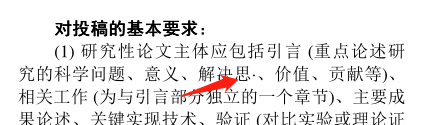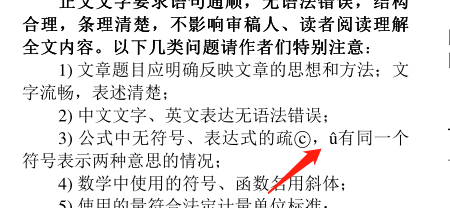换成texstudio+xelatex，按照网上配置中文的做法进行配置与修改，最终能够顺利编译，但依然无法正常显示中文。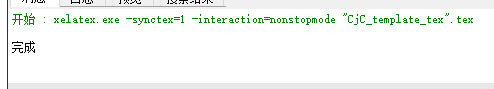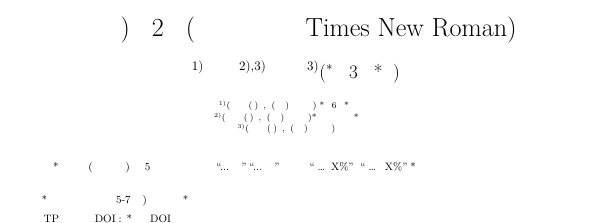最后换成texwork+miktex，按照网上做法配置与修改，并将模板中的gbk改为utf8，终于正常显示了。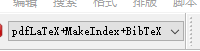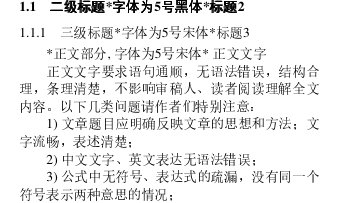现在还有一个问题不知如何解决：首页摘要较长情况下不会换页？


展开全文• LaTeX中文模板 先上代码 代码说明 编译结果   LaTeX中文模板   由于本人受够了mathtype的公式在word文字里面上蹿下跳甚至都显示不完整的尿性，决定入坑LaTeX。本人是初学者，用的是Ctex套装。可以官网...排版 Ctex
• 之前写了一份中文模板。但是标题摘要那一块趋于扁平化，没有比较酷的style。另外，如果用latex编译，是不支持除了eps之外的图片的插入。故而，做了这一份基于nips模板改进的模板。 首先，下载nips官方提供的模板，...nips pdflatex 插入
• 硕士博士顶级简约简历latex中文模板, 效果和使用注意事项，可以成功运行，请参考我的blog : https://blog.csdn.net/qq_41880787/article/details/108304527
• 中文模板https://blog.csdn.net/xueshengke/article/details/51996265?locationnum=2&fps=1亲测可直接使用，不过要把编译环境从pdflatex变为xeLatex,不然会报错“CTeX fontset `fandol’ is unavailable in ...
• 转载自 CTex官网 中科大学位论文模板 原文链接： http://www.ctex.org/PackageCASthesis 中科院学位论文宏包 CASthesis 宏包是以宏包作者的博士论文为基础模板，根据中国科学院研究生院学位论文撰写要求...
• 自用的latex中文模板，软件环境为texlive+texstudio。
• 基于最新LaTeX中文文档模板 基于最新LaTeX中文文档模板 基于最新LaTeX中文文档模板
• 带照片的latex中文简历模板，添加自己的经历后可以直接运行生成pdf，支持xelatex编译
• 好用的latex中文论文文档，是双栏文档，非常适合国内的一些主流期刊。。。。。。 只需要下载tex文件，然后在其中进行修改即可，值得注意的是，其中的一些依赖库可能到时候需要自己手动下一下
• IEEE的Latex中文模板，直接填充相应部分内容即可，方便好用，课程作业报告用了逼格陡升
• LaTeX笔记LaTeX简述LaTeX中文模板学习1.文章布局2.标题3.摘要、关键字4.正文4.1数学公式4.1.1数学公式的插入4.1.2 常用的数学公式4.2插入图片4.3插入表格4.3.1 一般的表格类型4.3.2 表格网格线的加粗5. 参考文献5.1 ...参考文献插入 插入表格
• ## Latex中文期刊论文模板

千次阅读 多人点赞 2020-07-13 21:25:27
Latex中文期刊论文模板 下载地址：CHN-PaperTemplate.rar 本模板基于DeathKing分享的中文模板完成。笔者在学习latex时使用的是刘海洋的书籍，而该中文模板在某些语句与刘海洋所授知识有出入，而且笔者无法重新编译...
• ## latex中文beamer模板

千次阅读 2019-05-23 11:11:06
%默认定义环境是不显示编号的，通过导言区使用开始使用定理编号 \setbeamertemplate{theorems}[numbered] %定理的标题显示标号 \theoremstyle{plain} %定理环境风格，plain是LaTeX的原始风格 %===================...beamer 参考文献
• 1. Latex使用的最全中文说明 《ChinaTeXMathFAQ_V1.1》.pdf 点击下载 2. Latex模板（可直接复制） 用tcolorbox设计一个漂亮的章节样式 戈亚斯联邦大学海报的主题模板
• 中文LaTeX简历模板 主要参考： 基本上是这个模版的魔改, 做了一些细小修改, 方便直接从overleaf编译. 使用步骤 Download ZIP Overleaf -> New Project -> Upload Project Do your own editing 选择complier为XeLaTeX
• ## LaTeX中文排版模板

千次阅读 2017-12-14 05:08:52
\XeTeXlinebreaklocale "zh" % 中文断行 % Define light and dark Microsoft blue colours \definecolor{MSBlue}{rgb}{.204,.353,.541} \definecolor{MSLightBlue}{rgb}{.31,.506,.741} % Define a new...
• \documentclass[options]{class} %文 档 类 声 明 \usepackage[options]{package} %引 入 宏 包 \begin{document} %正 ...自用文档模板 \documentclass[UTF8,10pt,a4paper]{article} \usepackage{geometry} \geomet
• 中文LaTeX处理模板 环境MiTex内核 编辑环境WinEdit 源码如下： \documentclass[a4paper,12pt]{article} \usepackage{CJK} %设定字号 \newcommand{\chuhao}{\fontsize{42pt}{\baselineskip}\selectfont} \new...
• ## Latex中文简历模板

万次阅读 2011-09-11 14:53:08
今天用Latex排版了一个中文的简历，感觉还行 采用的是moderncv的模板，所以需要 moderncv.cls文件（自己搜索一下吧），并且用Xelatex编译. 另有一个小问题不知如何修改，就是“基本信息”的上面有一行空白。 ...fortran fonts 算法 encoding
• 此资源已经重新上传，修正了若干由于latex版本升级造成的问题以及windows下的一些bug，完美支持中文，请大家到http://hhtao.weebly.com/download.html下载或者在csdn搜索同名资源已经标注修正字样！
• 在这里我们采用强大的latex编辑器进行简历排版,网上很多的Latex简历采用的是moderncv宏包进行制作,但是因为moderncv简历宏包是针对国外设计的,他的简历很多时候不符合我们的审美要求。本文采用全新设计的模板供大家...
• 非常漂亮的LaTex简历模板（著名的moderncv的中文版），有多种样式可供选择！使用XeLaTex进行编译，克服了使用CJK包时的种种中文化问题。建议使用CTexLive2009、texlive2010进行编译，注意必须使用xelatex命令编译。...
• 目录latex中文模板latex英文模板 latex中文模板 \documentclass[12pt,a4paper]{article} \usepackage[left=3.18cm, right=3.18cm, top=3.18cm, bottom=3.18cm]{geometry} \usepackage[UTF8]{ctex} %% \usepackage{......